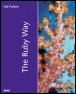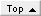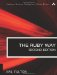# Accessing Environment VariablesRuby Way By Hal Fulton Slots : 1.0 Table of Contents

Occasionally we need to access environment variables as a link between our program and the outer world. An environment variable is essentially a label referring to a piece of text (typically a small piece); they can be used to store configuration information such as paths, usernames, and so on.

The notion of an environment variable is very common in the Unix world. The Windows world has borrowed it from Unix (by way of MS-DOS), so the code we show here should run on variants of both Windows and Unix.

#### Getting and Setting Environment Variables

The global constant ENV can be used as a hash both for purposes of retrieving and assigning values. Here we retrieve the value of an environment variable:

` `

` mypath = ENV["PATH"] # Let's get an array now... dirs = mypath.split(":") `

Here's an example of setting a variable. We take the trouble to fork another process to illustrate two facts. First of all, a child process inherits the environment variables that its parent knows. Second, an environment variable set by a child is not propagated back up to the parent.

` `

` ENV["alpha"] = "123" ENV["beta"]  = "456" puts "Parent: alpha = #{ ENV['alpha']} " puts "Parent: beta  = #{ ENV['beta']} " fork do   # Child code...   x = ENV["alpha"]   ENV["beta"] = "789"   y = ENV["beta"]   puts " Child: alpha = #{ x} "   puts " Child: beta  = #{ y} " end Process.wait a = ENV["alpha"] b = ENV["beta"] puts "Parent: alpha = #{ a} " puts "Parent: beta  = #{ b} " `

The output here would be the following:

` `

` Parent: alpha = 123 Parent: beta  = 456  Child: alpha = 123  Child: beta  = 789 Parent: alpha = 123 Parent: beta  = 456 `

There is a consequence of the fact that parent processes don't know about their children's variables. Because a Ruby program is typically run in a subshell, any variables changed during execution will not be reflected in the current shell after execution has terminated.

#### Storing Environment Variables as an Array or Hash

It's important to realize that ENV isn't really a hash; it just looks like one. For example, we can't call the invert method on it; it gives us a NameError because there is no such method. The reason for this implementation is the close tie between the ENV object and the underlying operating system; setting a value has an actual impact on the OS, a behavior that a mere hash can't mimic.

However, we can call the to_hash method to give us a real live hash:

` `

` envhash = ENV.to_hash val2var = envhash.invert `

Of course, once we have a hash, we can convert it to any other form we prefer (for example, an array):

` `

` envarr = ENV.to_hash.to_a `

It's not possible to directly reassign a hash to ENV; but we can fake it easily if we need to:

` `

` envhash = ENV.to_hash # Manipulate as needed... then assign back. envhash.each { |k,v| ENV[k] = v } `

#### Importing Environment Variables as Globals

A small library called importenv.rb will run through all the environment variables and import them into the program as global variables. It is used in this way:

` `

` require "importenv" # Now our environment variables are all globals... # E.g., \$PWD and \$LOGNAME where = \$PWD who = \$LOGNAME puts "In directory #{ where} , logged in as #{ who} " `

Note that because the importenv uses trace_var, the reflection is actually two-way: We can set one of these global variables in our program and the real environment variable will be set in the same way.

` `

` require "importenv" puts "My path is #\$PATH" # Prints: /usr/local/bin:/usr/bin:/usr/ucb:/etc:. \$PATH = "/ruby-1.8.0:" + \$PATH puts "My actual \$PATH variable is now #{ ENV['PATH']} " # Prints: /ruby-1.8.0:/usr/local/bin:/usr/bin:/usr/ucb:/etc:. `

Again, we point out that a change in an environment variable within a Ruby program doesn't affect the environment external to the program.The Ruby Way, Second Edition: Solutions and Techniques in Ruby Programming (2nd Edition)
ISBN: 0672328844
EAN: 2147483647
Year: 2000
Pages: 119
Authors: Hal Fulton

Similar book on Amazon